## Monday, May 30, 2011

### GAMS/Gurobi and Ctrl-C

I am seeing some strange results when I interrupt a GAMS/Gurobi run with Ctrl-c (in the IDE using the Interrupt button). The GAMS link should give in that case the best integer solution found so far, basically identical to an iteration or time limit. However the solution seems wrong: many of the binary variables x(i,j) in a large model are off (they are reported as zero instead of one). I suspect this is a problem in the GAMS link (i.e. not in Gurobi itself). From what I can see the link it passing back levels of integer variables incorrectly in these circumstances (I have seen this before: integer variables should have basis status SUPERBASIC to prevent only a basis status being sent back).

Btw, for this (huge) model the Gurobi heuristics do a fantastic job finding good solutions:

 Nodes    |    Current Node    |     Objective Bounds      |     Work Expl Unexpl |  Obj  Depth IntInf | Incumbent    BestBd   Gap | It/Node Time      0     0 179791.544    0 6744 1511067.07 179791.544  88.1%     -   91s H    0     0                    247836.12285 179791.544  27.5%     -   93s H    0     0                    232489.21120 179791.544  22.7%     -   96s      0     0 181272.276    0 7366 232489.211 181272.276  22.0%     -  397s H    0     0                    226736.43968 181272.276  20.1%     -  399s H    0     0                    218612.71830 181272.276  17.1%     -  404s

Just need to remember not to use the Interrupt button.

PS. Another minor issue with the link:

 MIP  Solution:        7259.007800    (-2147483648 iterations, 0 nodes) Best possible:        6916.876514 Absolute gap:          342.131286 Relative gap:            0.047132

it has troubles with the iteration count (which actually is 120570, a number that should not overflow).

## Thursday, May 19, 2011

Is turns out we also have to distribute x(t)’s such that we follow a non-uniform parameter. In the previous section http://yetanothermathprogrammingconsultant.blogspot.com/2011/05/optimal-spread.html we used a function v(t) = 1 with cumulative function w(t) = t.

In this case we consider a generic parameter v(t), with its cumulative cousin w(t)=w(t-1)+v(t). To trace this function as closely as possible we can use:E.g. when we use v(t)=t, then we see:

 ----     77 PARAMETER result                v           x i1        1.000 i2        2.000 i3        3.000 i4        4.000 i5        5.000       1.000 i6        6.000 i7        7.000 i8        8.000 i9        9.000       1.000 i10      10.000 i11      11.000 i12      12.000       1.000 i13      13.000 i14      14.000       1.000 i15      15.000 i16      16.000       1.000 i17      17.000 i18      18.000       1.000 i19      19.000 i20      20.000       1.000

## Saturday, May 14, 2011

In a multi-objective MIP model I am working I have a binary variable x(t). This variable is mostly zero but now and then it is one. One of the goals is to achieve an even spread of the ones. I.e.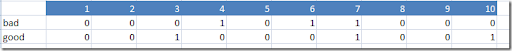The first row has the ones clustered in the middle. The second one looks pretty good.

The following idea is based on how Q-Q plots are used to find distributions in statistical data (http://en.wikipedia.org/wiki/Q-Q_plot).

First we form a running sum:

The “predicted’ running sum for an evenly distributed x(t) can be expressed as tn/T where n is the total number of ones, t is the current index and T is the last t:

Now we try to keep the values s(t) as close as possible to the predicted values tn/T. I.e.: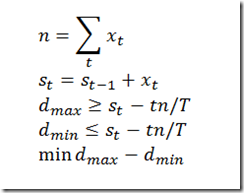Note that n is a variable in my model: I don’t know in advance how many x(t)’s are turned on. Indeed when I run this (with n=3) I see:

 ----     42 VARIABLE x.L  t3  1.000,    t7  1.000,    t10 1.000

Here are the results when we compare the two solutions (click to enlarge):Indeed this seems to confirm this approach could work, and we can add d(max)-d(min) as an extra objective to minimize.

## Wednesday, May 4, 2011

### Excel: Trace Dependents

I often use the “Trace Dependents” tool in Excel to quickly see which formulas use a given cell. Here is an example where I was a bit overwhelmed: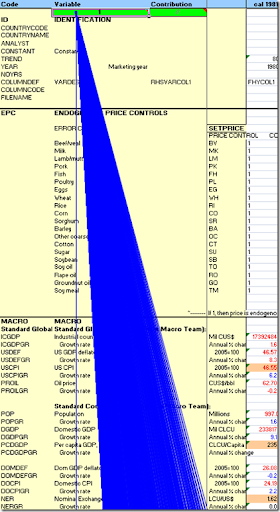## Tuesday, May 3, 2011

A spreadsheet model without circular references can be called “triangular”. Excel can form a dependency graph and ordering such that recalculation can be done in one iteration. If there are circular references the spreadsheet needs to perform more iterations before the results converge.

I was given a very large spreadsheet implementing an agricultural country trade model. The question was how near triangular is this? With the Excel tool to find circular references, we only find a single case:Luckily I have a tool that takes an Excel spreadsheet and parses Excel formulas and produces a GAMS representation of this. Essentially each cell forms an equation: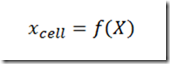The whole spreadsheet can be viewed as a fixed point expression: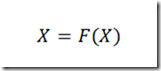This is of course just a system of nonlinear equations: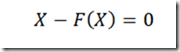When we solve this spreadsheet model as an NLP with Conopt we get some statistics:

 `---   67,618 rows  68,707 columns  232,364 non-zeroes ``---   776,017 nl-code  103,528 nl-non-zeroes` `   Iter Phase Ninf   Infeasibility   RGmax    NSB   Step InItr MX OK ``      0   0        7.3221660778E-07 (Input point) ``                                Pre-triangular equations:    22507 ``                                Post-triangular equations:   13897 ``      1   0        1.2425686171E-07 (After pre-processing) ``      2   0        3.4325009848E-11 (After scaling)`

Graphically, after reordering rows and columns, we have: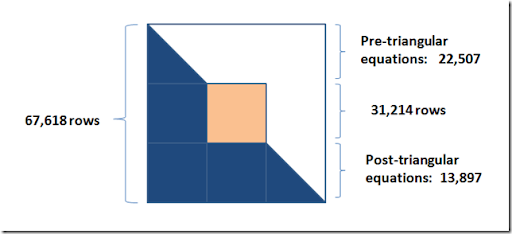This certainly gives an indication we are not close to a triangular model and we need a simultaneous equation solver to handle this type of model.

### Initial Point for NLP models

This example was introduced to illustrate the need for good initial points in NLP models (see http://amsterdamoptimization.com/pdf/snopt.pdf).

The problem is just a three variable model: find the smallest enclosing circle around n points. GAMS has a default initial point of zero which is often very bad. Only IPOPT can find an optimal solution quickly. But if we add a good starting point, all solvers find the optimal very quickly. Even the performance of IPOPT improves.

For a presentation I cleaned up the example a little bit: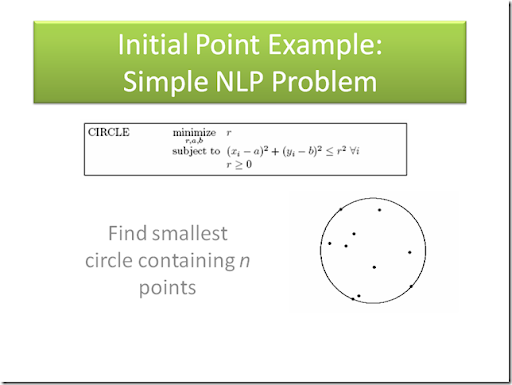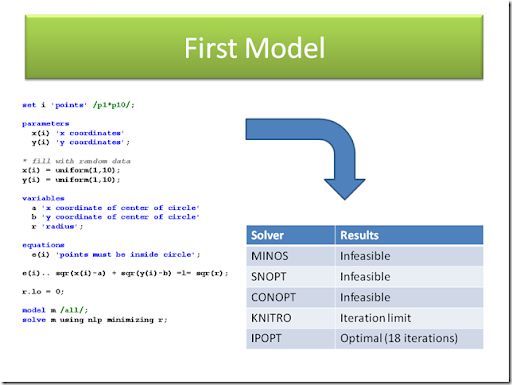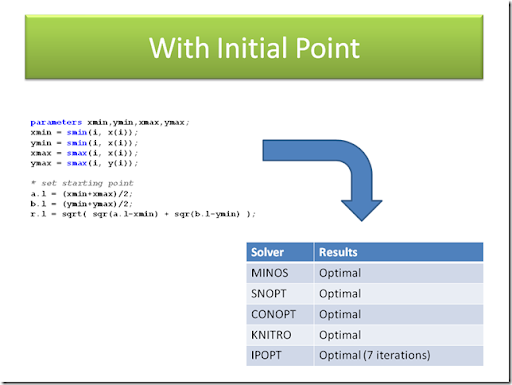Note: actually the model is easier to solve using a slightly different formulation: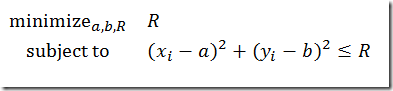(i.e. minimize the square of the radius r).